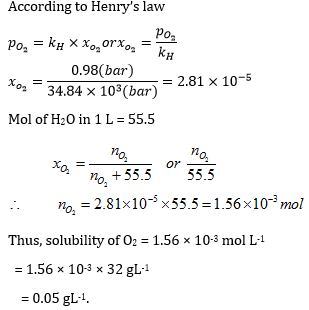# Henry's Law Formula

## Henry's Law Formulas and Examples

The law states that the solubility of an ideal gas in a liquid is directly proportional to the partial pressure of the gas over the liquid.

Mathematically,
Pαx
The units are correct. We rounded to one significant figure to match the number in the pressure. A 0.5-L bottle of cola has about 2 g (0.05 mol) of dissolved CO2.

Question: O2 is bubbled through water at 293 K. Assuming that O2 exerts a partial pressure of 0.98 bar. Calculate the solubility of O2 in gL-1. The value of Henry law constant (kH) for O2 is 34.84 k bar.
Options:
(a) 0.05 gL-1
(b) 0.08 gL-1
(c) 0.07 gL-1
(d) 0.01 gL-1